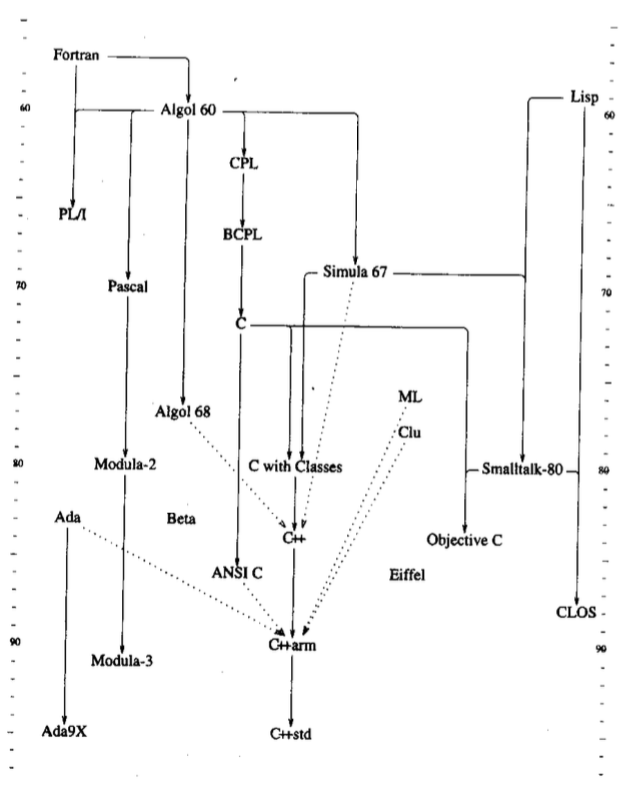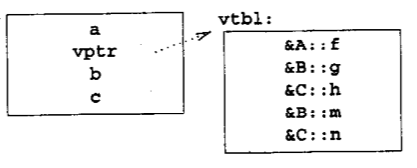# C++语言的设计与演化

## 第1章 C++的史前时代

### Simula

`Simula`的类能以协程`co-routine`的方式活动,很容易要求类的一个对象，与该类的其他对象以`伪并行`的方式工作。

`Simula`的类型系统的表达能力，以及编译系统捕捉类型错误的能力非常好。

C++的保护模型来自于访问权限许可、转让的概念；初始化与赋值的区分来自于对转让能力的思考；`const`概念从读写保护机制中演化出来的；异常处理来自有关容错系统的设计经历。

## 第2章 带类的C

`inline`提示编译器，将函数在调用处展开，而不是频繁调用，这提升了程序运行时的效率。

``````struct A { int x, y; };
struct B { int x, y; };``````

`Simula`提供了类、`Algol 68`提供了运算符重载、引用、以及在块里任何地方声明变量的能力、`Ada`提供了模板、异常。

### 运行时的保证

• 构建函数：建立起其他成员函数进行操作的环境基础。

• 析构函数: 销毁这个环境，并释放它以前获得的所有资源。

### 赋值的重载

`=`赋值的含义在C语言里语意是按位复制，对于自定义的类来说，肯定是不正确的。这种默认的赋值语义，实际上只是一种共享表示，而不是真正的副本。我对这个情况的反应，就是允许程序员自己描述类的复制意义。

``void X::operator=( Class X from ) { ... }``

## C++的诞生

C++出现的时间点。C++的用户是哪些人？

• 虚函数

• 函数名和运算符重载

• 引用

• 常量

• 用户可控制的自由空间存储区控制

• 改进的类型检查

### 虚函数

``````enum kind { circle, triangle, square };

class shape{
point center;
color col;
kind k; // k 是必须的，以便draw函数能确定在处理什么形状

public :
point where(){return center;}
void draw();
}

void shape::draw()
{
switch( k )
{
case circle:
// draw a circle
break;
case triangle:
// draw a triangle
break;
}
}``````

`Simula`的继承机制提供了一种解决方案：

• 首先需要提供一个类，用它定义所有形状的普遍性质

• 对那些必须针对每个特定的类型，专门实现的函数，标明为`virtual`

``````class shape{
point center;
color col;
public:
point where() { return center; };
virtual void draw();
}``````
• 如果要定义一个特定类型，我们必须先说明它是一个形状，然后再描述它的特殊类型。`circle`被说成由基类`shape`派生的。

``````class circle : public shape{
public:
void draw(){ ... };
void rotate(int) {};
}``````

``````class A{
int a;
public:
virtual void f();
virtual void g(int);
virtual void h(double);
}
class B : public A{
public :
int b;
void g(int); // 覆盖 A::g()
virtual void m(B*);
}
class C : public B{
public:
int c;
void h(double); // 覆盖 A::h()
virtual void n(C*);
}

void f( C* p )
{
p -> g(2);
}```````p->g(2)` 被编译后，就成了`(*(p->vptr)) ( p, 2 )`这样的间接调用形式。

### 基本重载

``````class complex{
double re, im;
public:
friend complex operator+( complex, complex );
}
complex z3 = z1 + z2; // operator+( z1, z2 )``````